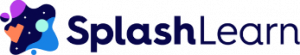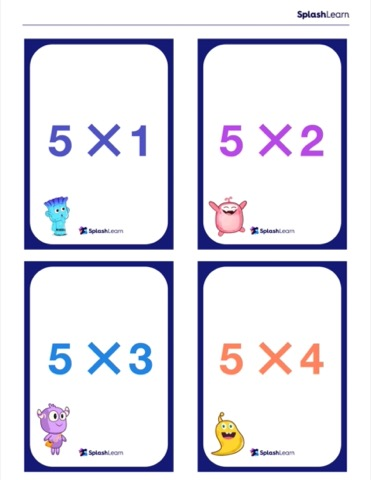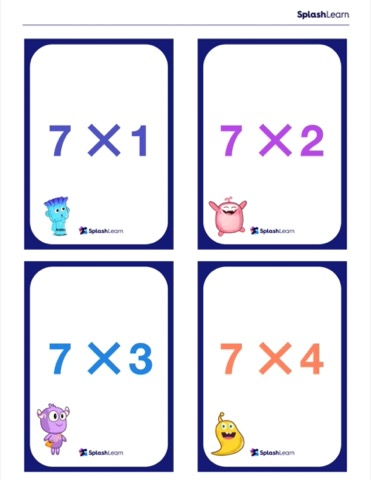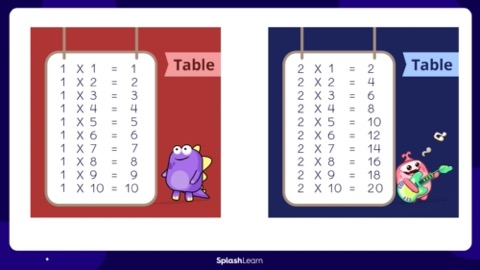More
BlogMath for KidsFree Printable Multiplication Chart and Times Tables

# Free Printable Multiplication Chart and Times Tables

Being a kid is not easy. There’s so much on their to-do list and so little time to execute it all. They have to remember the timings of their favorite shows and try to finish their greens. And mischief-making is an activity that requires careful planning on their part so that the pranks are funny enough to make someone laugh but not stupid enough for them to be grounded.

These are the things that come to mind when we see kids doing these activities. We don’t think about what pre or post-crisis analysis they do of their surroundings before coming up with their pranks. We may applaud their memory power when we see them recite dialogs from their favorite shows, but rarely do we take note of their observational skills when we see them mimic an animal. So, why do we become critical, and concerned, when it comes to their academic mastery?

The part where we want them to learn and grow academically is where we should be the most accommodating of our kid’s understanding prowess. It is where we should encourage them follow their instincts and to question their perspective. We should help them to solve problems their own way—by being smart and hitting the bullseye instead of shooting arrows in the dark.

We place much stress on practicing math because it makes our kids critical of the world around them and is important for their academic journey. But are we making sure that they practice the application of concepts and not simply memorize theories?

It’s true that concepts like addition and subtraction can be done on our fingers, but what about multiplication and division? Are they also easy enough to ingrain in a kid’s mind? Or do we need a more cumulative and engaging approach to teach our kids that it is not as difficult as it seems?

## SplashLearn: Most Comprehensive Learning Program for PreK-5SplashLearn inspires lifelong curiosity with its game-based PreK-5 learning program loved by over 40 million children. With over 4,000 fun games and activities, it’s the perfect balance of learning and play for your little one.

Kids don’t really put much thought into learning addition. They see a problem saying 2 + 1, they imagine two objects, conjure one more of the same type, count them all, and voila! 2 + 1 = 3.

But it is difficult for them to imagine objects in a similar way when they see an expression that has a cross instead of the plus sign.

2 ✕ 3 . . .

So what happens now?

Making multiplication fun!

We learned multiplication through a traditional, more conventional and boring approach called rote memorization. We just memorize facts of 2, answer the question and move on, thinking that we have fully understood the concept. But this becomes a problem when we encounter the concept in later grades, where multiplication is not just an independent problem to solve.

In such instances, multiplication concepts are connected to division concepts. And division gets interlinked with fractions, which are in turn connected to decimals. There’s an entire dynasty of concepts where kids cannot avoid multiplication because it has its own applications like estimating, evaluating amounts of money, order of operations, calculating distances, and even elapsed time problems.

So how do we make multiplication more approachable for kids and not just a saga of rote learning?

The answer lies in how we teach it at an elementary level. A simple term called Groups and an easy approach called Repeated Addition, are great places to start!

`Related Reading: New Math Tips & Strategies to Improve Kids’ Problems-Solving Skills`

## How to Start Teaching Multiplication: A Visual Approach – Groups

It’s important to introduce the concept of multiplication to kids the right way. The transition from addition to multiplication can be made easier with the help of “Groups”.

Let’s put it this way: using Groups is like sugarcoating multiplication, because this approach helps kids find it easier to understand. Perhaps then they won’t be quite as afraid of it.

The above image can easily be described as “5 groups of 2 airplanes each”.
And how do you find the total number of airplanes? By adding “2”, five times, i.e.,
2 + 2 + 2 + 2 + 2 and that is 10.

Easy!

## Repeated Addition to Multiplication Expressions

Once your little learners have aced writing repeated addition sentences, the next step is to teach them about multiplication expressions. Let’s consider this example:

4 groups of 5 turtles

5 + 5 + 5 + 5

Now, once your kid gets tired of writing addition expressions, you can introduce to them an easy way to write these expressions in a shorter and more concise manner. It won’t be difficult to let your kid observe that we have just written the number “5”, four times. Therefore, we can also write this as:

4 times 5

And this becomes the multiplication expression:

4 ✕ 5

But this step becomes crucial as this is where we subconsciously end up making a mistake. We start teaching them how to solve these expressions and forget about the fact that this step also needs to be practiced more.

Support Is Key

Before starting with skip counting, it’s imperative to bring a sense of familiarity with multiplication expressions.

Kids should be able to find the product without knowing multiplication rules. We can do this by encouraging learners to relate expressions to situations by giving them random multiplication expressions and asking them to imagine what may have resulted in this expression.

Allow their creativity to be a part of their learning experience. When all of this is done and your kids have stopped drawing their multiplication symbols, like the letter “X” of the alphabet (yes, there is a difference), you will know that they are ready!

Try this game and help your kid become an expert at turning repeated addition expressions into multiplication expressions before we move on to teaching them how to solve the expressions.

## Multiplication Strategies 101:

### Multiplying Using Fingers

We usually just add using fingers because it seems pretty easy and straightforward, but we can also multiply using fingers.

Let’s try to solve this expression:

4 ✕ 5

This strategy seems to make it easy to find the answer to a multiplication expression with smaller numbers.
PS: Don’t worry, we’ve got you covered! The next part deals with the strategy to multiply bigger numbers.

Step 1: Hold up as many fingers as the second number, which in this expression is the number 5.

Step 2: Skip count by the first number ( “4” in this case) as many times as the number of fingers you have raised.

And just like that, you have the answer to the given expression.

4 ✕ 5 = 20

### Distributive Property

Kids become comfortable with the multiplication facts of 2, 3, 4, 5 very easily and we can put this to good use by teaching them the distributive property aka the ultimate multiplication strategy.

Let’s try solving this expression:

3 ✕ 9

Step 1: Write the bigger number as the sum of 5 and another number:

3 ✕ 9 = 3 ✕ (5 + 4)

Step 2: Split this expression as a sum of two smaller expressions, like this:

3 ✕ 9 = (3 ✕ 5) + (3 ✕ 4)

Step 3: Solve these smaller expressions and add the resultants to find the final answer.

3 ✕ 9 = 15 + 12 = 27

Voila!

Three easy steps and our young learners will no longer ho-hum about multiplication.

Multiplication in Everyday Life

Multiplication strategies are more like simple rituals that can help kids solve problems. But this still won’t give them an idea of how important it is in the real world.

We can help our budding mathematicians associate multiplication to real life by asking them to check the next grocery bill that’s brought home or asking them about the number of saplings/flowers in a flower bed when they go gardening. Counting amounts of money while queueing at the cashier’s counter in a store where it’s a buy or bye situation, multiplication can be their savior in a real sense!

The process to prepare kids for higher grades begins once they have understood what multiplication is. For this purpose, there exists a very useful tool called the Multiplication Chart.

Let’s see how it works.

## Multiplication Chart

A basic multiplication chart contains multiplication facts of numbers from 1 to 10 and using a multiplication chart is as easy as making a sandwich. It could be even easier than that.

Let’s try solving this expression using the multiplication chart:

Equation: 7 ✕ 9

Step 1: Locate the 7th row and the 9th column.

Step 2: Look for the box where the row number 7 overlaps with column number 9.

And there we have it, the answer to our expression:

7 ✕ 9 = 63

To make things easier, you can refer to the multiplication chart given as a free printable at the end of this blog. We have also included an empty multiplication chart for you to make your own games like multiplication bingo!

## Commutative Property

Before you ask, multiplication charts do work the opposite way as well. You could look for the column that shows facts of 7 and the row that shows facts of 9 and the answer would still be the same.

This fact is referred to as the commutative property of multiplication i.e.,
7 ✕ 9 = 9 ✕ 7

The principle behind this property is as easy as it can get. It’s merely a play on perspective!

Take a look,

This picture shows 3 groups of 6 balls each, so the multiplication expression for this would be:
3 ✕ 6

But if we were to look at the picture another way,

Now it’s 6 groups of 3 balls each, so the multiplication expression would be:
6 ✕ 3

As the total number of balls is the same in any case, both the above expressions will have the same result.

So,

3 ✕ 6 = 6 ✕ 3

Kids can use this escape hatch when they are stuck on the times table of a bigger number and can effortlessly find the answer by recalling the related multiplication fact of a smaller number.

## Relevance of Multiplication Charts

Multiplication charts are important because they help students become familiar with the facts of different numbers. So, when our young learners move on to learning their times tables, it would not just be rote memorization. They would instead work up their brains to use commutative properties if they ever get stuck somewhere or they could also use the distributive property to derive a multiplication fact.

Using a multiplication chart is a stepping stone towards learning the process of multi-digit multiplication. As kids progress through different grades, multiplication becomes an essential and basic skill for the complicated aspects of math heading their way.

Thus, it is necessary for kids to be able to build upon what they already know and a multiplication chart can help them get comfortable with it.

Multiplication charts are scaffolding techniques that can help them learn in a better manner. But the true test is when kids start learning their times tables and we’re here to help with that!

## Times Tables

Times tables surely take us back to the most anxious days of our childhood. Kids establish a strong foothold in the math world once they accept that the times tables for 7s and 8s are not to be taken lightly.

2s, 5s, and 10s are called the brotherhood (or sisterhood) of multiplication as they never betray us. These numbers can help kids remember the other times tables as well.

Patience is the ultimate virtue to practice with little kids. If you show them a pattern in something, they readily accept that knowledge because it feels comfortable. Showing them a string of numbers and asking them to etch it in their memory will not help them in the long run.

Learning times tables is not something that can be achieved by just repeating it 3 times a day for 3 days. That sounds more like a prescription from the doctor than a strategy to learn something well. Nevertheless, here are some tips to help our learners with their times tables.

```Related Reading: Best Math Apps for Kids
```

## Tips for Times Tables Mastery

### Times Table of 1

This times table isn’t anything to be afraid of. Whatever number you multiply with 1, the result is the number itself.

### Times Table of 2

The number 2 is what we call “double or nothing!”

Any number you multiply with 2 will get doubled or will simply get added to itself.

### Times Table of 3

Here is the easiest trick to practice the times tables of 3. If you want to multiply a number by 3, multiply it by 2 first and then add the same number to it.

Let’s have a look at this example:

If you want to find 3 ✕ 6, go back to your 2s and check 2 ✕ 6 and add 6 to it.

So – 3 ✕ 6 = (2 ✕ 6 ) + 6 = 12 + 6 = 18

If you want to find 3 ✕ 8, go back to your 2s and check 2 ✕ 8, then add 8 to it.

So – 3 ✕ 8 = (2 ✕ 8) + 8 = 16 + 8 = 24

### Times Table of 4

Time for doubling the doubles. There is no easy way out of this one. If you want to multiply a number by 4, double it once, and then double what you get!

### Times Table of 5

The second in the beloved brotherhood, this number does not even need a trick, but if your kid still gets stuck with this one. Here is what you can do:

Any number that you want to multiply 5 with, attach 0 at its end and then half it!

For example,

If you want to find 5 ✕ 7, attach a 0 at the end of 7,

So now it’s 70. And half of 70 is 35.

So, 5 ✕ 7 = 35

### Times Table of 6

This one works just like the table of 3. If you want to find the product of a number with 6, go back to your 5s, multiply that number with 5 and then add the same number.

So, if you want to find 6 ✕ 8, do 5 ✕ 8 instead and then add 8 to it.

So 6 ✕ 8 = (5 ✕ 8 ) + 8 = 40 + 8 = 48.

### Times Table of 7

Before kids try to tackle this one, make sure they know their previous tables so this one does not seem as difficult.

Let’s see how we can approach this one.

Up until 7 times 6, the trick is to use the commutative property, i.e.

7 ✕ 2 = 2 ✕ 7 = 14

7 ✕ 3 = 3 ✕ 7 = 21

7 ✕ 4 = 4 ✕ 7 = 28

7 ✕ 5 = 5 ✕ 7 = 35

7 ✕ 6 = 6 ✕ 7 = 42

For the remaining numbers, the easiest trick is to remember to keep adding a group of 7.

7 ✕ 7 will be one more group of 7 than 7 ✕ 6,

So 7 ✕ 7 = 42 + 7 = 49

7 ✕ 8 will be one more group of 7 than 7 ✕ 7,

So 7 ✕ 8 = 49 + 7 = 56

And 7 ✕ 9 will be one more group of 7 than 7 ✕ 8

So 7 ✕ 9 = 56 + 7 = 63.

Tada! We have mastered the table of 7 as well.

### Times Table of 8

The easiest way to learn the times table of 8 is to use the commutative property for the first few steps and then adding a group of “8” for the remaining multiples, just like in your times table of 7.

For practice on this one, you can download flashcards for the times table of 8 attached at the end.

### Times Table of 9

An easy way to remember this times table is to use the facts of 10.

To multiply a number with 9, attach a zero at the end of the number and then subtract the same number.

For example,

To find 9 ✕ 8,

Attach a 0 at the end of 8 and subtract 8 from this;
That is, 9 ✕ 8 = 80 – 8 = 72

### Times Table of 10

The eldest and the easiest, kids can write this times table with their eyes closed and make no mistakes.
Just attach a zero at the end of whatever number you are multiplying with 10 and you have your answer.

10 ✕ 7 = 70

10 ✕ 10 = 100.

Time to pat yourself on the back!

Before you teach your fearless learner all about multiplication, we do have some resources that we think will be very useful when your kid begins this new adventure of understanding multiplication and its mysteries.

## Resources on Multiplication (Free printables)

For best practice and brushing up on all concepts of Multiplication, try these Multiplication Worksheets on SplashLearn.

You can also click on any of the below links to directly download these resources by SplashLearn.

`Related Reading: How to Help Kids with Math: 6 Innovating Approaches`### Multiplication Chart### Flash Card of 2### Flash Card of 3### Flash Card of 4### Flash Card of 5### Flash Card of 6### Flash Card of 7### Flash Card of 8### Flash Card of 9## Q1. How can I memorize things faster?

By practicing repeatedly, we can easily build reflex memory responses. For example, memorizing 6 ✕ 7 = 42 can be made easier by practicing the sentence, “six sevens are forty-two.”

## Q2. What are some times table games?

A great times tables game is “guess the expressions”, where the organizer says a number and you write down as many expressions as you can in a limited amount of time. The product of those expressions should be the said number. The person with the most correct answers wins.

## Q3. How do you teach multiplication to struggling students?

Use the method of skip counting or using fingers to multiply. Allow the student to practice repeated addition by drawing models of groups or arrays before they start learning their times tables. Learning about the commutative and distributive properties can be an added help.

## Q4. Are multiplication charts helpful?

It’s important to make students comfortable with a concept before they start practicing that concept on their own. Multiplication charts can be a good reference tool for practicing facts before starting the times table memorization process.

## Q5. What is the easiest way to learn multiplication?

The way we introduce a concept to a student is how they build their foundation of it. Asking the students to calculate double or triple of something can be fun, and it is a good starting point. Then we can gradually introduce the concept of groups or times tables when the students are at ease with the concept.

AUTHORAndrew Scholl
Andrew Scholl is an educational expert with over 15 years of teaching experience in elementary and middle school classrooms. He currently lives in New Jersey with his wife and two daughters.